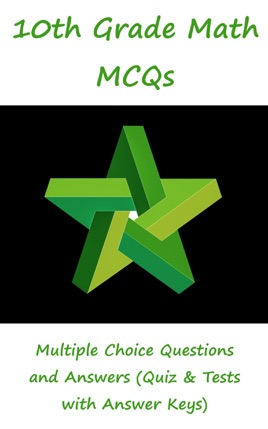• \$5.99

## Publisher Description

10th grade math multiple choice questions has 330 MCQs. Grade 10 math quiz questions and answers pdf, MCQs on matrices, algebraic expressions, angles, circular sector, basic operations on algebraic fractions, binary relation, circles, co terminal angles, complex roots, frequency polygon, histograms, cylinder, discriminant, absolute value, polygons, sets, factorization, simultaneous linear equations, frequency distribution, HCF and LCM MCQs and quiz are to practice exam prep tests.
10th grade math multiple choice quiz questions and answers pdf, math exam revision and study guide with practice tests for online exam prep and interviews. Math interview questions and answers to ask, to prepare and to study for jobs interviews and career MCQs with answers keys.
3rd, 4th, mean and continued quiz has 9 multiple choice questions. Algebraic formulas and applications quiz has 24 multiple choice questions. Algebraic manipulation quiz has 13 multiple choice questions. Basic statistics quiz has 28 multiple choice questions. Factorization quiz has 7 multiple choice questions. General math quiz has 26 multiple choice questions.
Geometry quiz has 42 multiple choice questions. Introduction to trigonometry quiz has 41 multiple choice questions. Linear equations and inequalities quiz has 25 multiple choice questions and answers. Matrices and determinants quiz has 47 multiple choice questions. Ratio, proportions and variations quiz has 21 multiple choice questions and answers. Roots of quadratic equations quiz has 24 multiple choice questions with answers. Sets and functions quiz has 24 multiple choice questions.
Math jobs' interview questions and answers pdf, MCQs on solution of quadratic equation and simultaneous linear equations, solving linear inequalities, square root of algebraic expression, surds and their applications, third proportional, triangle, trigonometric identities, trigonometric ratios, types of matrices, addition and subtraction of matrices, algebraic expressions, angles, area of circular sector, basic concepts, basic operations on algebraic fractions, binary relation, circle's basic concepts, circles, co terminal angles, complex cube roots of unity, construction of frequency polygon, construction of histograms, continued proportional, cylinder, degree, discriminant, equations involving absolute value, escribed circle, example of sets, factorization of expressions, formulae, fourth proportional, fraction, frequency distribution, H.C.F and L.C.M, improper fraction, introduction, joint variation, k method, linear equations, linear inequalities, math exam questions, math theorems, matrix, mean proportional, measurement of angle, measures of central tendency, measures of dispersion, multiplication of matrices, multiplicative inverse, multiplicative inverse of matrix, ordered pairs, parallel lines, polygon, proper fraction, proportion, quadrants, quadratic equation, radian measure of angle, radical equation, radius of circle, ratio, rational fraction, reciprocal equation, reciprocal identities, recognition of operations on sets, rectangle, relation between radians and degree, relation between roots and co-efficient, sector of circle, sets, variation MCQs based worksheets for competitive exams preparation.